# AP Chemistry Chapter 8 Sample Exercises

Jane Hamze
7 de Jun de 2010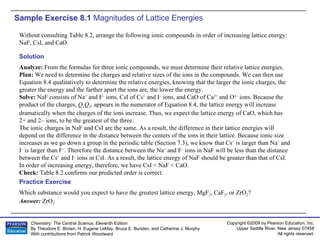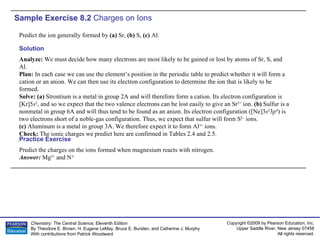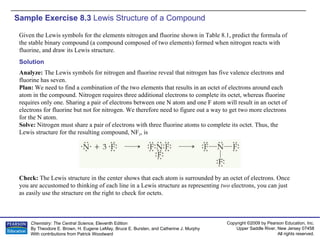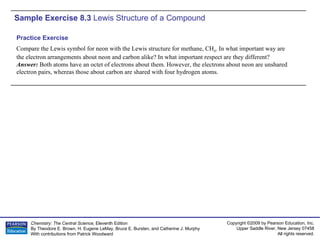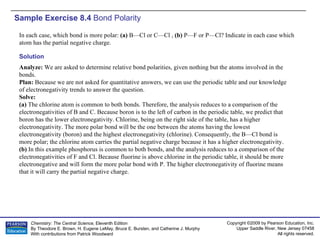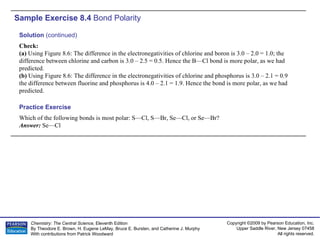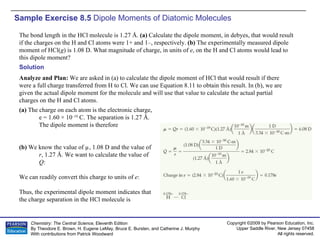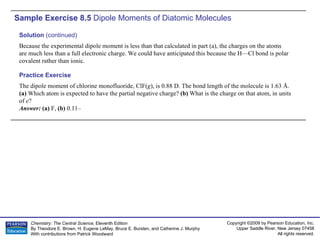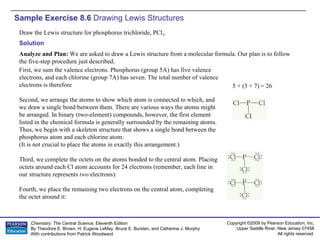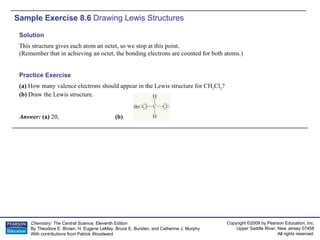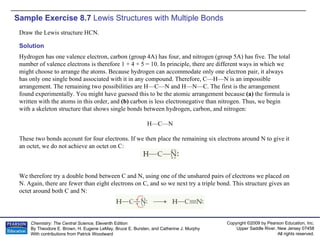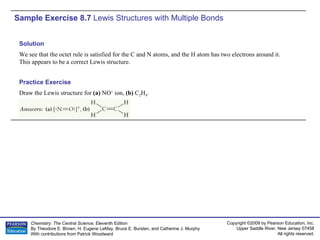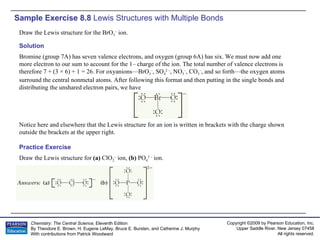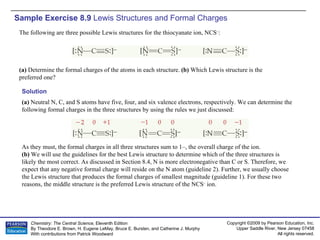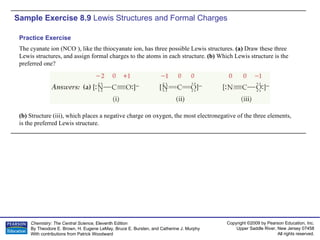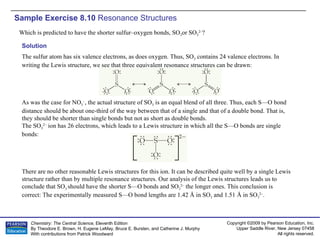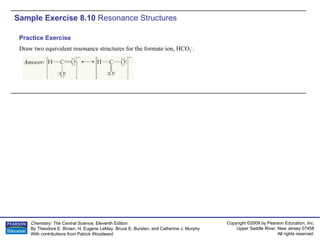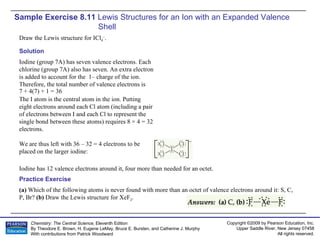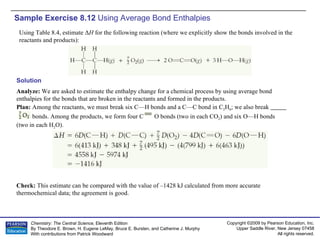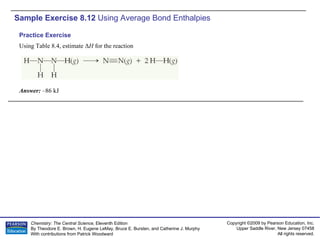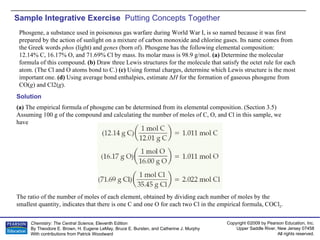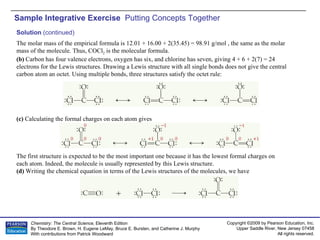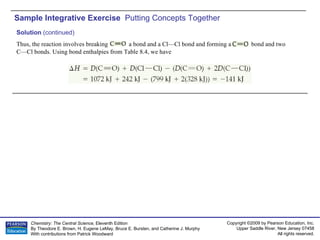1 de 23

### AP Chemistry Chapter 8 Sample Exercises

• 1. Sample Exercise 8.1 Magnitudes of Lattice Energies Without consulting Table 8.2, arrange the following ionic compounds in order of increasing lattice energy: NaF, CsI, and CaO. Solution Analyze: From the formulas for three ionic compounds, we must determine their relative lattice energies. Plan: We need to determine the charges and relative sizes of the ions in the compounds. We can then use Equation 8.4 qualitatively to determine the relative energies, knowing that the larger the ionic charges, the greater the energy and the farther apart the ions are, the lower the energy. Solve: NaF consists of Na + and F – ions, CsI of Cs + and I – ions, and CaO of Ca 2+ and O 2– ions. Because the product of the charges, Q 1 Q 2 , appears in the numerator of Equation 8.4, the lattice energy will increase dramatically when the charges of the ions increase. Thus, we expect the lattice energy of CaO, which has 2+ and 2– ions, to be the greatest of the three. The ionic charges in NaF and CsI are the same. As a result, the difference in their lattice energies will depend on the difference in the distance between the centers of the ions in their lattice. Because ionic size increases as we go down a group in the periodic table (Section 7.3), we know that Cs + is larger than Na + and I – is larger than F – . Therefore the distance between the Na + and F – ions in NaF will be less than the distance between the Cs + and I – ions in CsI. As a result, the lattice energy of NaF should be greater than that of CsI. In order of increasing energy, therefore, we have CsI < NaF < CaO. Check: Table 8.2 confirms our predicted order is correct. Which substance would you expect to have the greatest lattice energy, MgF 2 , CaF 2 , or ZrO 2 ? Answer: ZrO 2 Practice Exercise
• 2. Sample Exercise 8.2 Charges on Ions Predict the ion generally formed by (a) Sr, (b) S, (c) Al. Predict the charges on the ions formed when magnesium reacts with nitrogen. Answer: Mg 2+ and N 3– Practice Exercise Solution Analyze: We must decide how many electrons are most likely to be gained or lost by atoms of Sr, S, and Al. Plan: In each case we can use the element’s position in the periodic table to predict whether it will form a cation or an anion. We can then use its electron configuration to determine the ion that is likely to be formed. Solve: (a) Strontium is a metal in group 2A and will therefore form a cation. Its electron configuration is [Kr]5 s 2 , and so we expect that the two valence electrons can be lost easily to give an Sr 2+ ion. (b) Sulfur is a nonmetal in group 6A and will thus tend to be found as an anion. Its electron configuration ([Ne]3 s 2 3 p 4 ) is two electrons short of a noble-gas configuration. Thus, we expect that sulfur will form S 2– ions. (c) Aluminum is a metal in group 3A. We therefore expect it to form Al 3+ ions. Check: The ionic charges we predict here are confirmed in Tables 2.4 and 2.5.
• 3. Sample Exercise 8.3 Lewis Structure of a Compound Given the Lewis symbols for the elements nitrogen and fluorine shown in Table 8.1, predict the formula of the stable binary compound (a compound composed of two elements) formed when nitrogen reacts with fluorine, and draw its Lewis structure. Solution Analyze: The Lewis symbols for nitrogen and fluorine reveal that nitrogen has five valence electrons and fluorine has seven. Plan: We need to find a combination of the two elements that results in an octet of electrons around each atom in the compound. Nitrogen requires three additional electrons to complete its octet, whereas fluorine requires only one. Sharing a pair of electrons between one N atom and one F atom will result in an octet of electrons for fluorine but not for nitrogen. We therefore need to figure out a way to get two more electrons for the N atom. Solve: Nitrogen must share a pair of electrons with three fluorine atoms to complete its octet. Thus, the Lewis structure for the resulting compound, NF 3 , is Check: The Lewis structure in the center shows that each atom is surrounded by an octet of electrons. Once you are accustomed to thinking of each line in a Lewis structure as representing two electrons, you can just as easily use the structure on the right to check for octets.
• 4. Sample Exercise 8.3 Lewis Structure of a Compound Compare the Lewis symbol for neon with the Lewis structure for methane, CH 4 . In what important way are the electron arrangements about neon and carbon alike? In what important respect are they different? Answer: Both atoms have an octet of electrons about them. However, the electrons about neon are unshared electron pairs, whereas those about carbon are shared with four hydrogen atoms. Practice Exercise
• 5. Sample Exercise 8.4 Bond Polarity In each case, which bond is more polar: (a) B—Cl or C—Cl , (b) P—F or P—Cl? Indicate in each case which atom has the partial negative charge. Solution Analyze: We are asked to determine relative bond polarities, given nothing but the atoms involved in the bonds. Plan: Because we are not asked for quantitative answers, we can use the periodic table and our knowledge of electronegativity trends to answer the question. Solve: (a) The chlorine atom is common to both bonds. Therefore, the analysis reduces to a comparison of the electronegativities of B and C. Because boron is to the left of carbon in the periodic table, we predict that boron has the lower electronegativity. Chlorine, being on the right side of the table, has a higher electronegativity. The more polar bond will be the one between the atoms having the lowest electronegativity (boron) and the highest electronegativity (chlorine). Consequently, the B—Cl bond is more polar; the chlorine atom carries the partial negative charge because it has a higher electronegativity. (b) In this example phosphorus is common to both bonds, and the analysis reduces to a comparison of the electronegativities of F and Cl. Because fluorine is above chlorine in the periodic table, it should be more electronegative and will form the more polar bond with P. The higher electronegativity of fluorine means that it will carry the partial negative charge.
• 6. Sample Exercise 8.4 Bond Polarity Which of the following bonds is most polar: S—Cl, S—Br, Se—Cl, or Se—Br? Answer: Se—Cl Practice Exercise Solution (continued) Check: (a) Using Figure 8.6: The difference in the electronegativities of chlorine and boron is 3.0 – 2.0 = 1.0; the difference between chlorine and carbon is 3.0 – 2.5 = 0.5. Hence the B—Cl bond is more polar, as we had predicted. (b) Using Figure 8.6: The difference in the electronegativities of chlorine and phosphorus is 3.0 – 2.1 = 0.9 the difference between fluorine and phosphorus is 4.0 – 2.1 = 1.9. Hence the bond is more polar, as we had predicted.
• 7. Sample Exercise 8.5 Dipole Moments of Diatomic Molecules The bond length in the HCl molecule is 1.27 Å. (a) Calculate the dipole moment, in debyes, that would result if the charges on the H and Cl atoms were 1+ and 1–, respectively. (b) The experimentally measured dipole moment of HCl( g ) is 1.08 D. What magnitude of charge, in units of e , on the H and Cl atoms would lead to this dipole moment? Solution Analyze and Plan: We are asked in (a) to calculate the dipole moment of HCl that would result if there were a full charge transferred from H to Cl. We can use Equation 8.11 to obtain this result. In (b), we are given the actual dipole moment for the molecule and will use that value to calculate the actual partial charges on the H and Cl atoms. (a) The charge on each atom is the electronic charge, e = 1.60 × 10 –19 C. The separation is 1.27 Å. The dipole moment is therefore (b) We know the value of µ, 1.08 D and the value of r , 1.27 Å. We want to calculate the value of Q : We can readily convert this charge to units of e : Thus, the experimental dipole moment indicates that the charge separation in the HCl molecule is
• 8. Sample Exercise 8.5 Dipole Moments of Diatomic Molecules The dipole moment of chlorine monofluoride, ClF( g ), is 0.88 D. The bond length of the molecule is 1.63 Å. (a) Which atom is expected to have the partial negative charge? (b) What is the charge on that atom, in units of e ? Answer: (a) F, (b) 0.11– Practice Exercise Solution (continued) Because the experimental dipole moment is less than that calculated in part (a), the charges on the atoms are much less than a full electronic charge. We could have anticipated this because the H—Cl bond is polar covalent rather than ionic.
• 9. Sample Exercise 8.6 Drawing Lewis Structures Draw the Lewis structure for phosphorus trichloride, PCl 3 . Solution Analyze and Plan: We are asked to draw a Lewis structure from a molecular formula. Our plan is to follow the five-step procedure just described. First, we sum the valence electrons. Phosphorus (group 5A) has five valence electrons, and each chlorine (group 7A) has seven. The total number of valence electrons is therefore Second, we arrange the atoms to show which atom is connected to which, and we draw a single bond between them. There are various ways the atoms might be arranged. In binary (two-element) compounds, however, the first element listed in the chemical formula is generally surrounded by the remaining atoms. Thus, we begin with a skeleton structure that shows a single bond between the phosphorus atom and each chlorine atom: (It is not crucial to place the atoms in exactly this arrangement.) Third, we complete the octets on the atoms bonded to the central atom. Placing octets around each Cl atom accounts for 24 electrons (remember, each line in our structure represents two electrons): Fourth, we place the remaining two electrons on the central atom, completing the octet around it: 5 + (3 × 7) = 26
• 10. Sample Exercise 8.6 Drawing Lewis Structures Solution This structure gives each atom an octet, so we stop at this point. (Remember that in achieving an octet, the bonding electrons are counted for both atoms.) (a) How many valence electrons should appear in the Lewis structure for CH 2 Cl 2 ? (b) Draw the Lewis structure. Answer: (a) 20, (b) Practice Exercise
• 11. Sample Exercise 8.7 Lewis Structures with Multiple Bonds Draw the Lewis structure HCN. Solution Hydrogen has one valence electron, carbon (group 4A) has four, and nitrogen (group 5A) has five. The total number of valence electrons is therefore 1 + 4 + 5 = 10. In principle, there are different ways in which we might choose to arrange the atoms. Because hydrogen can accommodate only one electron pair, it always has only one single bond associated with it in any compound. Therefore, C—H—N is an impossible arrangement. The remaining two possibilities are H—C—N and H—N—C. The first is the arrangement found experimentally. You might have guessed this to be the atomic arrangement because (a) the formula is written with the atoms in this order, and (b) carbon is less electronegative than nitrogen. Thus, we begin with a skeleton structure that shows single bonds between hydrogen, carbon, and nitrogen: H—C—N These two bonds account for four electrons. If we then place the remaining six electrons around N to give it an octet, we do not achieve an octet on C: We therefore try a double bond between C and N, using one of the unshared pairs of electrons we placed on N. Again, there are fewer than eight electrons on C, and so we next try a triple bond. This structure gives an octet around both C and N:
• 12. Sample Exercise 8.7 Lewis Structures with Multiple Bonds Solution We see that the octet rule is satisfied for the C and N atoms, and the H atom has two electrons around it. This appears to be a correct Lewis structure. Draw the Lewis structure for (a) NO + ion, (b) C 2 H 4 . Practice Exercise
• 13. Sample Exercise 8.8 Lewis Structures with Multiple Bonds Draw the Lewis structure for the BrO 3 – ion. Solution Bromine (group 7A) has seven valence electrons, and oxygen (group 6A) has six. We must now add one more electron to our sum to account for the 1– charge of the ion. The total number of valence electrons is therefore 7 + (3 × 6) + 1 = 26. For oxyanions—BrO 3 – , SO 4 2 – , NO 3 – , CO 3 – , and so forth—the oxygen atoms surround the central nonmetal atoms. After following this format and then putting in the single bonds and distributing the unshared electron pairs, we have Notice here and elsewhere that the Lewis structure for an ion is written in brackets with the charge shown outside the brackets at the upper right. Draw the Lewis structure for (a) ClO 2 – ion, (b) PO 4 3 – ion. Practice Exercise
• 14. Sample Exercise 8.9 Lewis Structures and Formal Charges The following are three possible Lewis structures for the thiocyanate ion, NCS – : (a) Determine the formal charges of the atoms in each structure. (b) Which Lewis structure is the preferred one? Solution (a) Neutral N, C, and S atoms have five, four, and six valence electrons, respectively. We can determine the following formal charges in the three structures by using the rules we just discussed: As they must, the formal charges in all three structures sum to 1–, the overall charge of the ion. (b) We will use the guidelines for the best Lewis structure to determine which of the three structures is likely the most correct. As discussed in Section 8.4, N is more electronegative than C or S. Therefore, we expect that any negative formal charge will reside on the N atom (guideline 2). Further, we usually choose the Lewis structure that produces the formal charges of smallest magnitude (guideline 1). For these two reasons, the middle structure is the preferred Lewis structure of the NCS – ion.
• 15. Sample Exercise 8.9 Lewis Structures and Formal Charges The cyanate ion (NCO – ), like the thiocyanate ion, has three possible Lewis structures. (a) Draw these three Lewis structures, and assign formal charges to the atoms in each structure. (b) Which Lewis structure is the preferred one? (b) Structure (iii), which places a negative charge on oxygen, the most electronegative of the three elements, is the preferred Lewis structure. Practice Exercise
• 16. Sample Exercise 8.10 Resonance Structures Which is predicted to have the shorter sulfur–oxygen bonds, SO 3 or SO 3 2– ? Solution The sulfur atom has six valence electrons, as does oxygen. Thus, SO 3 contains 24 valence electrons. In writing the Lewis structure, we see that three equivalent resonance structures can be drawn: As was the case for NO 3 – , the actual structure of SO 3 is an equal blend of all three. Thus, each S—O bond distance should be about one-third of the way between that of a single and that of a double bond. That is, they should be shorter than single bonds but not as short as double bonds. The SO 3 2– ion has 26 electrons, which leads to a Lewis structure in which all the S—O bonds are single bonds: There are no other reasonable Lewis structures for this ion. It can be described quite well by a single Lewis structure rather than by multiple resonance structures. Our analysis of the Lewis structures leads us to conclude that SO 3 should have the shorter S—O bonds and SO 3 2– the longer ones. This conclusion is correct: The experimentally measured S—O bond lengths are 1.42 Å in SO 3 and 1.51 Å in SO 3 2– .
• 17. Sample Exercise 8.10 Resonance Structures Draw two equivalent resonance structures for the formate ion, HCO 2 – . Practice Exercise
• 18. Sample Exercise 8.11 Lewis Structures for an Ion with an Expanded Valence Shell Draw the Lewis structure for ICl 4 – . Iodine has 12 valence electrons around it, four more than needed for an octet. Solution Iodine (group 7A) has seven valence electrons. Each chlorine (group 7A) also has seven. An extra electron is added to account for the 1– charge of the ion. Therefore, the total number of valence electrons is The I atom is the central atom in the ion. Putting eight electrons around each Cl atom (including a pair of electrons between I and each Cl to represent the single bond between these atoms) requires 8 × 4 = 32 electrons. We are thus left with 36 – 32 = 4 electrons to be placed on the larger iodine: 7 + 4(7) + 1 = 36 (a) Which of the following atoms is never found with more than an octet of valence electrons around it: S, C, P, Br? (b) Draw the Lewis structure for XeF 2 . Practice Exercise
• 19. Sample Exercise 8.12 Using Average Bond Enthalpies Using Table 8.4, estimate Δ H for the following reaction (where we explicitly show the bonds involved in the reactants and products): Solution Analyze: We are asked to estimate the enthalpy change for a chemical process by using average bond enthalpies for the bonds that are broken in the reactants and formed in the products. Plan: Among the reactants, we must break six C—H bonds and a C—C bond in C 2 H 6 ; we also break bonds. Among the products, we form four C O bonds (two in each CO 2 ) and six O—H bonds (two in each H 2 O). Check: This estimate can be compared with the value of –1428 kJ calculated from more accurate thermochemical data; the agreement is good.
• 20. Sample Exercise 8.12 Using Average Bond Enthalpies Using Table 8.4, estimate Δ H for the reaction Answer: –86 kJ Practice Exercise
• 21. Sample Integrative Exercise Putting Concepts Together Phosgene, a substance used in poisonous gas warfare during World War I, is so named because it was first prepared by the action of sunlight on a mixture of carbon monoxide and chlorine gases. Its name comes from the Greek words phos (light) and genes (born of). Phosgene has the following elemental composition: 12.14% C, 16.17% O, and 71.69% Cl by mass. Its molar mass is 98.9 g/mol. (a) Determine the molecular formula of this compound. (b) Draw three Lewis structures for the molecule that satisfy the octet rule for each atom. (The Cl and O atoms bond to C.) (c) Using formal charges, determine which Lewis structure is the most important one. (d) Using average bond enthalpies, estimate Δ H for the formation of gaseous phosgene from CO( g ) and Cl2( g ). Solution (a) The empirical formula of phosgene can be determined from its elemental composition. (Section 3.5) Assuming 100 g of the compound and calculating the number of moles of C, O, and Cl in this sample, we have The ratio of the number of moles of each element, obtained by dividing each number of moles by the smallest quantity, indicates that there is one C and one O for each two Cl in the empirical formula, COCl 2 .
• 22. Sample Integrative Exercise Putting Concepts Together Solution (continued) The molar mass of the empirical formula is 12.01 + 16.00 + 2(35.45) = 98.91 g/mol , the same as the molar mass of the molecule. Thus, COCl 2 is the molecular formula. (b) Carbon has four valence electrons, oxygen has six, and chlorine has seven, giving 4 + 6 + 2(7) = 24 electrons for the Lewis structures. Drawing a Lewis structure with all single bonds does not give the central carbon atom an octet. Using multiple bonds, three structures satisfy the octet rule: (c) Calculating the formal charges on each atom gives The first structure is expected to be the most important one because it has the lowest formal charges on each atom. Indeed, the molecule is usually represented by this Lewis structure. (d) Writing the chemical equation in terms of the Lewis structures of the molecules, we have
• 23. Sample Integrative Exercise Putting Concepts Together Solution (continued) Thus, the reaction involves breaking a bond and a Cl—Cl bond and forming a bond and two C—Cl bonds. Using bond enthalpies from Table 8.4, we have